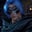Related Tags

julia
communitycreator
arrays

# What are arrays in Julia?Gutha Vamsi Krishna

In this shot, we will learn about arrays in Julia.

An array is an ordered collection of items.

In Julia, we can create arrays that hold multiple types of values, or we can restrict them to hold a single type of value.

There are two types of arrays in Julia:

• One-dimensional arrays: These arrays have only one dimension.
• Multidimensional arrays: These arrays have two or more dimensions.
Representation of arrays in Julia

### Syntax

We can declare a one-dimensional array either like this:

array_name = type_of_array[value1, value2, value3]


Or this:

array_name = [value1, value2, value3]


where some examples of type_of_array are Int64, Float64, and so on, and comma-separated values are provided between the square brackets [].

We can declare a two-dimensional array either like this:

array_name = type_of_array[value1, value2, value3 ; value4, value5, value6]


Or this:

array_name = [value1, value2, value3 ; value4, value5, value6]


where values are separated by a space and every row is separated using a semicolon (;).

### Example of a one-dimensional array

In the following example, we are creating a one-dimensional array, integer_array, which is of type Int64. This means that we are restricting it to store only values of type Int64. In the example, we are also creating a string array named string_array and storing string values in it.

#declare and initialize the single dimensional array
integer_array = Int64[1000,2000, 3000, 4000]
print(integer_array)
print("\n")
print(string_array)

### Example of a two-dimensional array

In the following example, we are creating a two-dimensional array of type Int64, where each of the values are separated by a space and all of the rows are separated by semicolons (;).

#declare and initialize the two dimensional array
integer_array = Int64[1000 2000 3000 ; 4000 5000 6000]

#use display to pretty print the 2d array
display(integer_array)

### Access an element

In Julia, the index of arrays starts from 1, unlike in other languages where the index starts from 0.

We can access any element by providing its index between square brackets.

### The syntax for a one-dimensional array

array_name[index_of_the_element]


### The syntax for a two-dimensional array

array_name[row][column]


We can access a two-dimensional array using its row and column indices.

Let’s look at an example of how we can access an element from an array in Julia:

Representation of array indices in Julia
#declare and initialize the single dimensional array
integer_array_1d = Int64[1000, 2000, 3000, 4000]

#access element at second postion
println(integer_array_1d)

#declare and initialize the two dimensional array
integer_array_2d = Int64[1000 2000 3000 ; 4000 5000 6000]

#access element at second row, first column
println(integer_array_2d)

RELATED TAGS

julia
communitycreator
arrays

CONTRIBUTORGutha Vamsi Krishna
RELATED COURSES

View all Courses

Keep Exploring

Learn in-demand tech skills in half the time